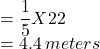## At what meter mark will Ario be when Miguel starts the race? Round to the nearest tenth. x = (StartFraction m Over m + n EndFraction) (x 2 m

Question

At what meter mark will Ario be when Miguel starts the race? Round to the nearest tenth. x = (StartFraction m Over m + n EndFraction) (x 2 minus x 1) + x 1 A number line goes from 0 to 25. A line is drawn from 3 to 25. The point at 3 is labeled Start and the point at 25 is labeled End. Miguel and his brother Ario are both standing 3 meters from one side of a 25-meter pool when they decide to race. Miguel offers Ario a head start. Miguel says he will start when the ratio of Arioâ€™s completed meters to Arioâ€™s remaining meters is 1:4. 4.4 meters 7.4 meters 17.6 meters 20.6 meters

in progress 0
2 months 2021-07-29T21:09:39+00:00 2 Answers 4 views 0

(A)4.4 Meters

Explanation:

• The point at 3 is labeled Start and the point at 25 is labeled End.
• Since, they start at the 3 meters mark, distance to be covered

=25-3=22 meters

Miguel says he will start when the ratio of Ario’s completed meters to Ario’s remaining meters is 1:4.

Since the distance is 22 Meters

The point at which Miguel will start the race2. Answer: the correct answer is actually 7.4 meters I just took it and got a 100

Explanation: